Courses

# NCERT Exemplar: Nuclei- 2 Notes | EduRev

## JEE : NCERT Exemplar: Nuclei- 2 Notes | EduRev

The document NCERT Exemplar: Nuclei- 2 Notes | EduRev is a part of the JEE Course JEE Revision Notes.
All you need of JEE at this link: JEE

Q.16. Why do stable nuclei never have more protons than neutrons?
Ans.
The reason is that protons, being charged particles, repel each other. This repulsion becomes so great in nuclei with more than 10 protons or so, that an excess of neutrons which produce only attractive forces, is required for stability.
Important point: As you get to heavier elements, with each new proton you add, there is a larger repulsive force. The nuclear force is attractive and stronger than the electrostatic force, but it has a finite range. So you need to add extra neutrons, which do not repel each other, to add extra attractive force. You eventually reach a point where the nucleus is just too big, and tends to decay via alpha decay or spontaneous fission.
To view this in quantum mechanical terms, the proton potential well is not as deep as the neutron well due to the electrostatic repulsion. [Due to the Pauli exclusion principle, you only get two particles per level (spin up and spin down)]. If one well is filled higher than the other, you tend to get a beta decay to even them out. As the nuclei get larger, the neutron well gels deeper as compared to the proton well and you get more neutrons than protons.

Q.17. Consider a radioactive nucleus A which decays to a stable nucleus C through the following sequence:
A → B → C
Here B is an intermediate nuclei which is also radioactive.
Considering that there are N0 atoms of A initially, plot the graph showing the variation of number of atoms of A and B versus time.
Ans.
Consider radioactive nucleus A have N0 atoms of A initially; or at t = 0, NA = N0 (maximum) whole NB = 0. As time increases, NA decreases exponentially and the number of atoms of B increases. After some time NB becomes maximum. As B is an intermediate nuclei which is also radioactive, it also start decaying and finally drop to zero exponentially by radioactive decay law. We can represent the situation as shown in the graph.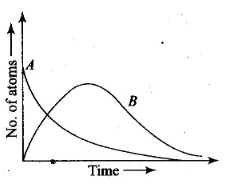Q.18. A piece of wood from the ruins of an ancient building was found to have a 14C activity of 12 disintegrations per minute per gram of its carbon content. The 14C activity of the living wood is 16 disintegrations per minute per gram. How long ago did the tree, from which the wooden sample came, die? Given half-life of 14C is 5760 years.
Ans.
Rate of disintegration in old wood sample of C-14 radioactive atoms is 12 atoms per min gm. Initially rate of disintegration of C-14 when the tree was live=16 atoms per min per gm.
T1/2 of C-14 nuclei=5760 years
N=N0e-λt or R=R0e-λt
12=16e-λt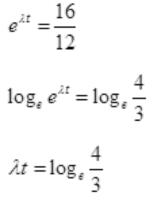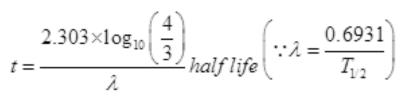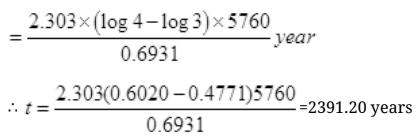Q.19. Are the nucleons fundamental particles, or do they consist of still smaller parts? One way to find out is to probe a nucleon just as Rutherford probed an atom. What should be the kinetic energy of an electron for it to be able to probe a nucleon? Assume the diameter of a nucleon to be approximately 10–15 m.
Ans.
To detect the properties of nucleons inside the nucleus the wavelength of particle which may detect nucleons that must be of size of nucleons (10-15 m). So the wavelength of particle which can detect the nucleons must be equal to or less than 10-15
λ = 10-15 m
λ = h/p
∵ E = hv = hc/λ    [∵c = vλ]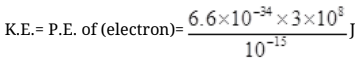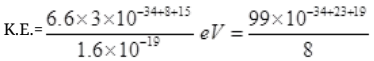=12.4×10-34+42=1.24×101×10+8
K.E. = 1.24×109eV
So the K.E. of particle which may detect nucleon inside the nucleus must be of 1.24×10eV per particle.

Q.20. A nuclide 1 is said to be the mirror isobar of nuclide 2 if Z1 =N2 and Z2 =N1 .
(a) What nuclide is a mirror isobar of 2311Na ?
(b) Which nuclide out of the two mirror isobars have greater binding energy and why?
Ans.

Here Z is atomic number and N is no. of neutron in 11Na23
Z1=11
N1=23-11=12
Mirror isobar of 11Na23 is
Z= N= 12
So Mg is isobar of 11Na23
So 12Mg23 is the mirror isobar of 11Na23
(b) As the neutrons in 12Mg23 are ‘11’ and in 11Na23 are ‘12’ so, the number of neutrons in Na is larger than Mg and hence nuclear short range attractive forces in Na will be larger than repulsive electrostatic forces between proton-proton.
So, 11Na23 has more binding energy than 12Mg23

Q.21. Sometimes a radioactive nucleus decays into a nucleus which itself is radioactive. An example is: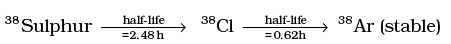Assume that we start with 1000 38S nuclei at time t = 0. The number of 38Cl is of count zero at t = 0 and will again be zero at t =∞ . At what value of t, would the number of counts be a maximum?
Ans.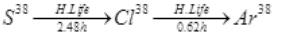Initially at t=0, number of radioactive atoms of S38 = N1 and of Cl38 are zero.
At any time t,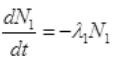and N1=N0e1t
It is the rate of formation of Cl38 from S38. Let N2 is the number of of Cl38 atoms (radioactive):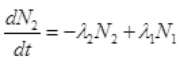1N0e1t2N2    ...(I)
Multiplying both sides by e2t dt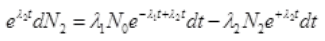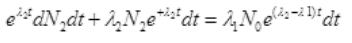Integrating both sides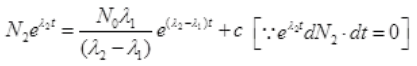∴ Cl38 atom is formed after disintegration of S38, so initially number of Cl38 atoms are N2=0.
at t=0, N2=0,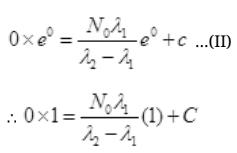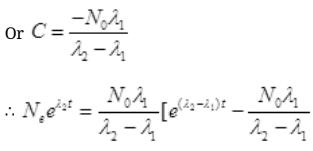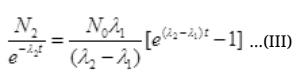Multiplying E2t to both sides we get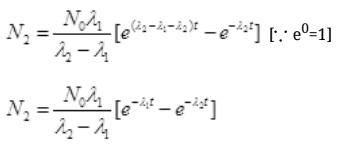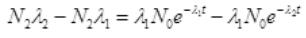Nare the number of S38 atoms
No. Are Cl38 atoms after time ti will be N2=N0e2t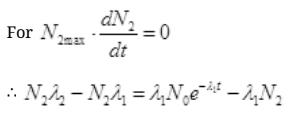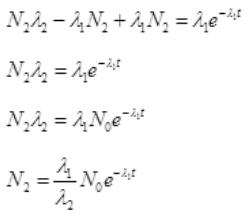Put the value of N2 in (III)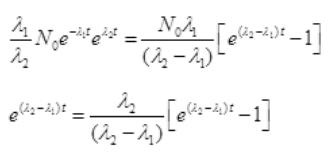By cross multiplication and multiplying both sides by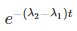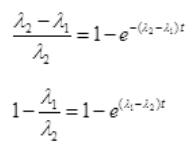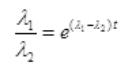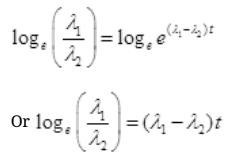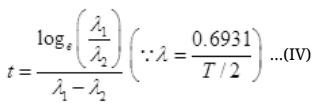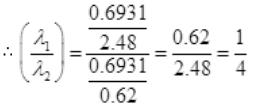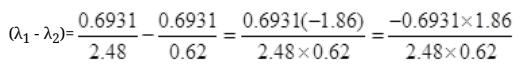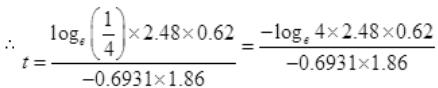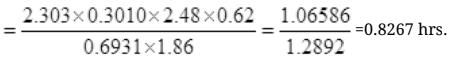Number Cl38 radioactive atoms will be maximum at N= 0.8267 hrs.

Q.22. Deuteron is a bound state of a neutron and a proton with a binding energy B = 2.2 MeV. A γ -ray of energy E is aimed at a deuteron nucleus to try to break it into a (neutron + proton) such that the n and p move in the direction of the incident γ-ray.
If E = B, show that this cannot happen. Hence calculate how much bigger than B must E be for such a process to happen.
Ans.
Binding energy (B) of deuteron = 2.2 MeV
Some part of energy of γ-ray is used up against binding energy B = 2.2 MeV and the rest part will impart K.E. to neutron and proton.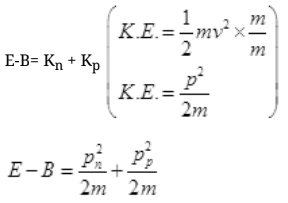By law of conservation of momentum,
∴ Pn + pp= momentum of γ-ray of Energy E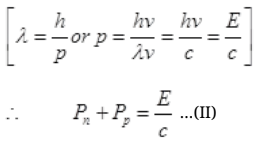Case I: If E=B then from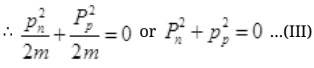It can be possible if pn= pp=0 because square of non zero number can never zero.
If pn=pp=0 then equation IInd cannot be satisfied and the process cannot take place. From II,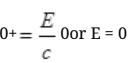but energy E of γ ray cannot be zero.
Case II: if E>B or E=B+λ where λ will be very small than B then from (I),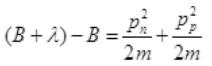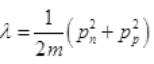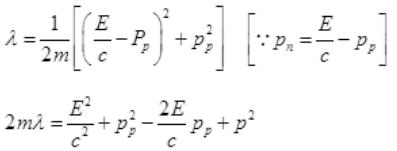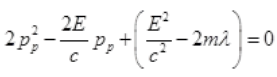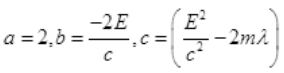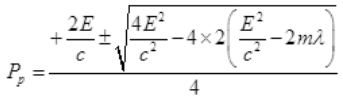For a real and equal value of Pp discriminant must be zero as the value of pp must be one.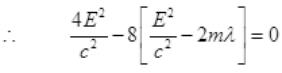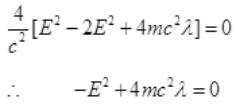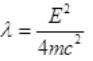∵ λ is very small
So E=B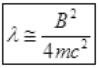Q.23. The deuteron is bound by nuclear forces just as H-atom is made up of p and e bound by electrostatic forces. If we consider the force between neutron and proton in deuteron as given in the form of a Coulomb potential but with an effective charge e′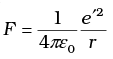estimate the value of (e’/e) given that the binding energy of a deuteron is 2.2 MeV.
Ans. The binding energy of H atom in ground state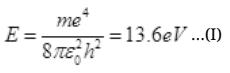If proton and neutron had charge e’ each and governed by the same electrostatic force, then in the above equation we would need to replace electronic mass m by the reduced mass m’ of proton-neutron (as some mass of proton and neutron is used by binding energy) and electronic charge e is replaced by e’.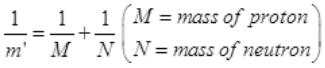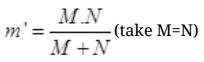=M/2 ( if m = mass of electron)
m' = 1836m/2 = 918 m
∴ Binding Energy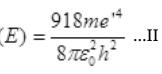Dividing (II) by (i) we get,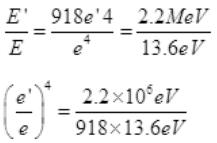e'/e = 2200000/1248.48 = (176.21)1/4
Required ratio e'/e = 3.64

Q.24. Before the neutrino hypothesis, the beta decay process was throught to be the transition,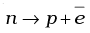If this was true, show that if the neutron was at rest, the proton and electron would emerge with fixed energies and calculate them.Experimentally, the electron energy was found to have a large range.
Ans.
Neutron was at rest before β decay from neutron. Hence energy of neutron=En=mncand momentum of neutron pn=0 as its velocity is zero.
By the law of conservation of momentum,
Pn=pp+ p(Beta)
O= pp+ pe
Let pe=pp then
⇒ |pp|=|pe|=p(eV)
Energy of proton = Ep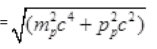Energy of electron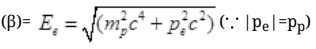From conservation,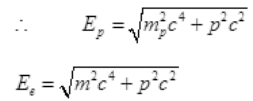∴ By the law of conservation of energy,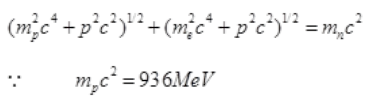and mnc2 =938 MeV
and mec2= 0.5 meV
As the energy difference in neutron and proton is very small, pc will be small pc<<mpcwhile pc may be greater than mec2 so by neglecting (mec2)2= (0.5)(given)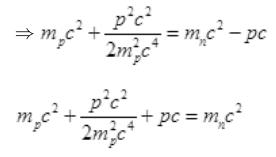Again pc<<mpc2 so neglecting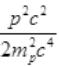we get
Pc = mnc2-mpc=938 MeV-936 MeV
Pc=2 MeV is the momentum
E=mc2
∵ E2 = m2c4
E is the energy of either proton or neutron then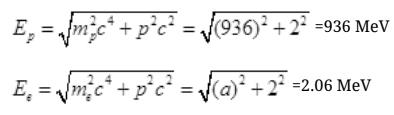Q.25. The activity R of an unknown radioactive nuclide is measured at hourly intervals. The results found are tabulated as follows: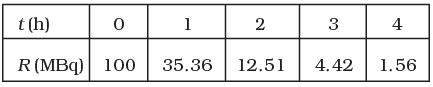(i) Plot the graph of R versus t and calculate half-life from the graph.
(ii) Plot the graph of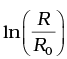versus t and obtain the value of half-life from the graph.
Ans.

(i) Graph between R versus t is exponential curve. From the graph at slightly more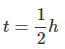the R should be 50% so at R =50% the  t(h)=0.7h
=0.7×60 min
=42 min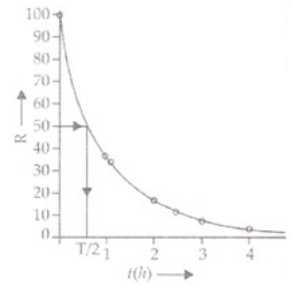(ii) For Graph between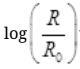versus t(h)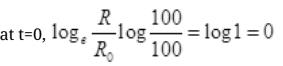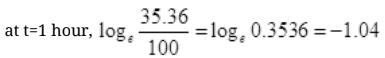=2.302 log10 0.3536=1.04
at t=2 hours,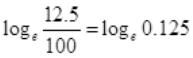= 2.303log10 0.125 = -2.08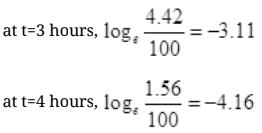t (hours) 1 2 3 4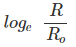-1.04 -2.08 -311 -4.16

The graph showing the variation ofversus t(h) as follows:
We know that disintegration constant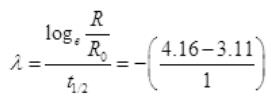λ = -1.05 per hour
t1/2 = 0.6931/λ = 0.6931/1.05
t1/2 =  42 min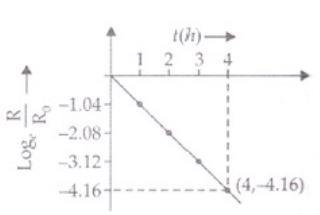Q.26. Nuclei with magic no. of proton Z = 2, 8, 20, 28, 50, 52 and magic no. of neutrons N = 2, 8, 20, 28, 50, 82 and 126 are found to be very stable.
(i) Verify this by calculating the proton separation energy Sp for 120Sn (Z = 50) and 121Sb = (Z = 51).
The proton separation energy for a nuclide is the minimum energy required to separate the least tightly bound proton from a nucleus of that nuclide. It is given by
Sp = (MZ–1, N + MH – MZ,N )c2.
Given 119In = 118.9058u, 120Sn = 119.902199u, 121Sb = 120.903824u, 1H = 1.0078252u.
(ii) What does the existence of magic number indicate?
Ans.

(i) Sp = (MZ–1, N + MH – MZ,N )c2
Here in this formula MZ-1 is the mass of atom of Z-1 atomic number.
Mis the mass of atom of mass number Z.
∴ MZ-1= Mass of atom whose atomic number is 50-1=49.
It is 49In119 in this case MZ-1 = 49In119 = 118.9058 and N=119 - 49 = 70.
Sp for 50Sn120=c2[118.9058+1.0078252-199.902199]
Sp for 50Sn120=0.0114362c2
Now for Sp of 51Sb121
Sp= [Mz-1, N+MH-Mz,N]c2
Z=51, Z-1=50 for Sn
MZ-1=mass of 50Sn=199.902199 u
∴ Sp for 51Sb121= [199.902199+1.0078252-120.903824]c2
= 0.0059912c2
∴ Sp(50Sn120)>Sp(51Sb121)
(ii) The existence of magic numbers indicates that the shell structure of nucleus is similar to the shell structure of atom. This also explains the peaks in binding energy per nucleon curve.

Offer running on EduRev: Apply code STAYHOME200 to get INR 200 off on our premium plan EduRev Infinity!

170 docs

,

,

,

,

,

,

,

,

,

,

,

,

,

,

,

,

,

,

,

,

,

;